# Concept of Current Transformer with Examples

This article is about Concept of Current Transformer with Examples and focusing to the engineers, technicians and supervisors. You will find lot of documents related to this article. Just navigate our website  www.paktechpoint.com  and find more articles. Please! Do not forget to subscribe our You tube channel also. Thanks in Advance.PLEASE SUBSCRIBE OUR PAKTECHPOINT YOUTUBE CHANNEL## Concept of Current Transformer with Examples

### Current Transformer (C.T.)

• In a current transformer it’s primary winding is connected in series with the line carrying the load current. The primary winding of CT consists of very few turns (usually 1). The secondary winding of CT has large number of turns which is determined by the secondary terminals.
• One of the terminals of the secondary winding of CT is earthed to project the equipment and personal in the event of an insulation breakdown in the current transformer.
• The schematic diagram of a current transformer is shown in below Figure with the load and burden (Ammeter, relay etc.) connected to the secondary winding of the C.T.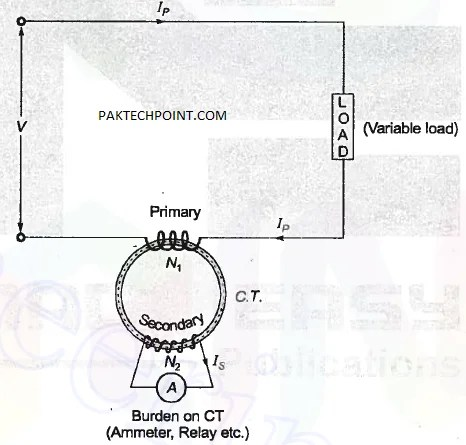### Ratio of Current Transformer(C.T.)

#### 1.Transformation Ratio:

it is the ratio of the magnitude of primary current phasor to the secondary current phasor.#### 2. Nominal Ratio:

It is the ratio of rated primary winding current to the rated secondary winding current.

Kn =Rated primary winding current / Rated secondary winding current

#### 3. Turns Ratio:

It is the ratio of number of turns of secondary winding to the number of turns of primary winding i.e.

NOTE:#### Ratings of Current Transformers

The primary winding current in a CT is high whereas the secondary winding Current is low. Therefore, it is rated as:

Remember 800/600/200(Primary current IP) 1A (Secondary current IS)

Popularly adopted rating = 200/1A

• A CT is used with its primary winding connected in series With the line carrying the current and therefore, the primary current depends upon the load connected to the system and is not determined by the load or the burden connected on the secondary winding of the CT.
• Is = (N1/N2) IP for reducing the secondary current, the number of turns on the secondary is increased.
• Primary winding of CT has only single turn. This is called “Bar primary”. It’s used to reduce the errors in the current transformer.

### Equivalent Circuit of a CT

Below Figure shows an equivalent circuit diagram of a current transformer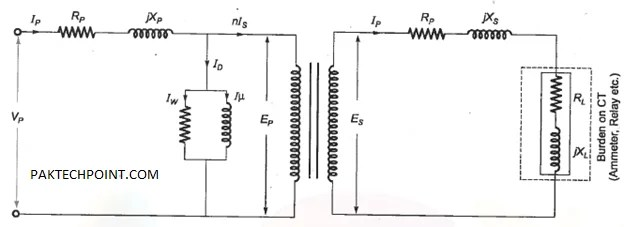And below Figure shows corresponding phasor diagram.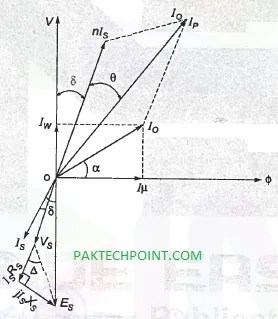Here, Rp = Primary winding resistance

Xp Primary winding reactance

Rs = Secondary winding resistance

Xs = Secondary winding resistance

Ip and is are primary and secondary currents respectively

IO = Exciting current

IM = Magnetizing component of exciting current

Iw = Core loss component of exciting current

Ep and Es are emf induced in the primary and secondary windings

Iµ = Magnetizing component of exciting current

Δ = Secondary load angle— tan-1= (XL/RL)Angle between Is and Es

Let the turns ratio of this CT be#### Errors in a CT

From the equivalent circuit diagram of CT, we haveTransformation ratio of CT=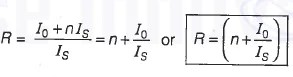The two types of error occurring in a CT are:

1. #### Ratio Error:

The exact primary to secondary current ratio of a CT is not equal to the turns ratio of the CT due to the no-load component of the current (IO), This causes the error in CT which is called a “ratio error”.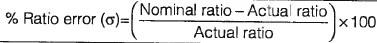Nominal ratio, Kn = n = Turns ratio

Actual ratio =R= IP/IS =(Primary current phasor /Secondary current phasor) Ratio error depends on no-load current of CT.

#### Phase angle error

The angle by which the secondary current phasor when reversed, differs in phase from the primary current is called the “phase angle error.The phase angle error also depends on the no-load current of CT.

Calculation of Ratio and Phase Angle Error of a CT

#### Ratio Error

Consider a section of phasor diagram of CT as shown in below Figure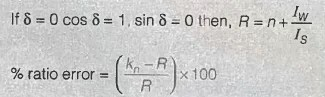The ratio error depends upon the no load current , IO and secondary load angle.

#### Phase angle Error (θ)

From phasor diagram we have,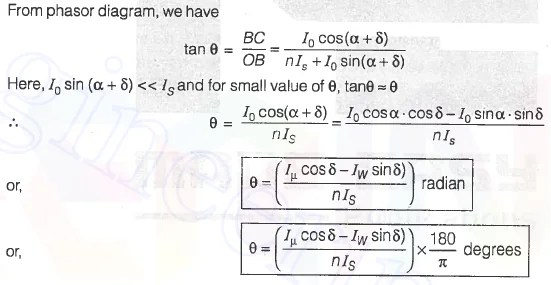NOTE: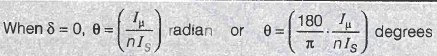The phase angle error depends on the no-load component current, Iw, Iµ and [wand secondary burden angle (θ).

### QUESTIONS AND ANSWERS FOR CURRENT TRANSFORMER

#### Example 1

A current transformer rated 100/5 has magnetizing and loss components of the exciting current as 1 A and 0.6 A respectively. The secondary winding burden is purely resistive. Its transformation ratio at rated current is

(a)20.2 (c) 20.12 (b) 200.2 (d) None of these

Solution: (c)

Given,

turns ratio of CT, n=100/5 = 20

Secondary rated current,    Is=5A

Magnetizing current,         Iµ=1A

Core loss component of exciting current,   Iw    = 0.6A

We know that, transformation ratio of CT,

R= n+( Iw    / Is) =20+(0.6/5)=20.12

Example 2

The current in the primary winding of a current transformer depends upon:

(a) Burden of the secondary winding of transformer

(b) Load connected to the system in which CT is installed

(c) Both burden of the transformer secondary winding and load connected to the system

(d) None of the above

Solution: (b)

Primary winding current only depends upon the load current.

Example 3

A current transformer has a rating of 1000/5. Its magnetizing current and loss component of exciting current are 10 A and 6 A respectively. The phase angle between secondary winding induced emf and current is 300. The phase angle error of the transformer at rated current is (a) 0.4960 (c) 0.650 (b) 0.5890 (d) 0.3240

Solution: (d)

Given, turns ratio,  n= 1000/5=200

Iµ= 10 A, IW= 6A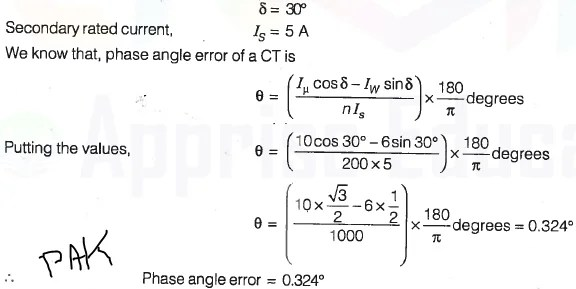Example 4

The advantages of instrument transformers are

(a) the readings of instruments used in conjunction with them do not depend upon their resistance, inductance etc.

(b) the ratings of instrument transformers have been standarized and the rating of instruments used in conjunction with them also get standardized. Therefore, there is reduction of cost and case in replacements.

(c) the metering circuit is electrically isolated from the power circuit thereby providing safety to operating personal.

(d) all of the above

Solution: d

Example 5

The nominal ratio of a current transformer is

(a) primary winding current/secondary winding current.

(b) rated primary winding current/rated secondary winding current.

(c) number of secondary winding turns/number of primary winding turns.

(d) all of the above

Solution: (b)

Nominal ratio (of C.T.), Rated primary winding current / Rated secondary winding current

Example 6

A CT is having a turns ratio of 1: 199 and rated as 1000/5A. 25 VA. The core loss and magnetizing component of the primary current are 4 and 7A under rated conditions. Determine the phase angle and ratio errors for the rated burden and rated secondary current at 0.8 pf lagging and 0.8 pf leading. Neglect the resistance and leakage reactance of secondary winding.

Solution: (a)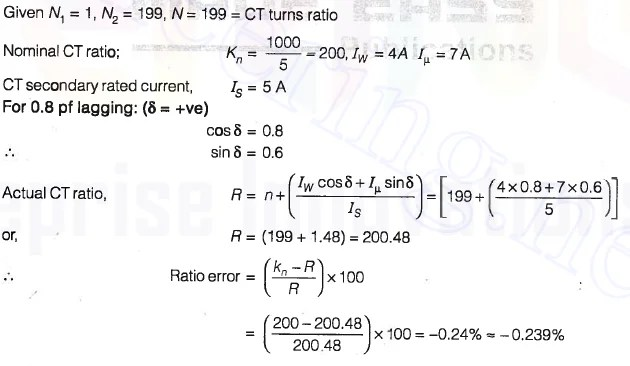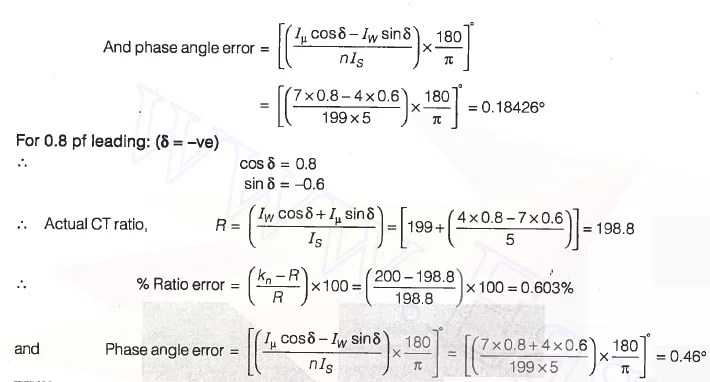### 2 Responses

1.folorentorium says:

I think other website proprietors should take this site as an model, very clean and fantastic user genial style and design, let alone the content. You are an expert in this topic!

2.privacy screens says:

Fantastic web site. Lots of useful info here. I am sending it to a few friends ans also sharing in delicious. And certainly, thanks for your effort!